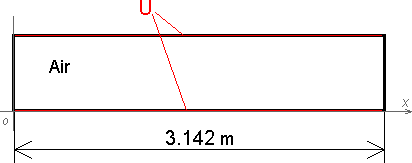QuickField

A new approach to field modelling
 Language: Global English Deutch Espanol Francais Italiano Danmark Ceske Chinese Pycckuü

>> >>

# Harmonic analysis of saw function

This example demonstrates the accuracy of the harmonic browser (included in QuickField package)
Rectangular wave duct consists of two electrodes. The electrodes carry electric potential in a form of saw-function. Perform Fourier analysis of electric potential distribution.

Problem type:

Plane-parallel problem of electrostatics.Given:

Relative permittivity of air ε = 1,
Boundary potentials - saw function.

Perform the Fourier analysis of the voltage distribution curve. Use the harmonic browser to calculate amplitudes of the harmonics and compare results with analytical solution.

Solution:

Voltage distribution along the X axis corresponds to the "saw" function.
U(0≤x<1.507) = 2·x
U(x=1.507) = 0
U(1.507<x≤3.142) = 2·x - 2·π

Fourier analysis of this "saw" function gives the following analytical solution:*
f(x) = 2 · ( sin(x) - sin(2x) / 2 + sin(3x) / 3 - ... + (-1)n+1·sin(nx) / n )

Results:
Potential distribution along the bottom boundaryHarmonic browsers windowHarmonic browser allows calculation of the first hundred harmonics.
 Harmonic Amplitude Error QuickField Theory 1 2 2 - 2 0.9999 1 - 3 0.6666 0.6666 - 4 0.4999 0.5 - 5 0.3999 0.4 - 10 0.1999 0.2 0.05% 20 0.0998 0.1 0.2% 50 0.0383 0.04 5% 100 0.0168 0.02 15%

*Mathematical Methods for Physicists. A Comprehensive Guide. 7th Edition. George Arfken, Hans Weber, Frank Harris, p 937-938. ISBN 978-0-12-384654-9.

• Video:
• View simulation report in PDF
• Download simulation files (files may be viewed using any QuickField Edition).